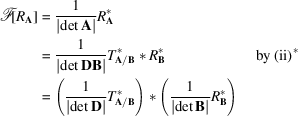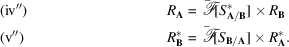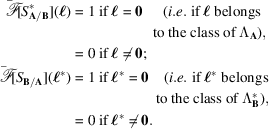International
Tables for
Crystallography
Volume B
Reciprocal space
Edited by U. Shmueli

International Tables for Crystallography (2010). Vol. B, ch. 1.3, pp. 48-49   | 1 | 2 |

## Section 1.3.2.7.2.4. Relation between Fourier transforms

G. Bricognea

aGlobal Phasing Ltd, Sheraton House, Suites 14–16, Castle Park, Cambridge CB3 0AX, England, and LURE, Bâtiment 209D, Université Paris-Sud, 91405 Orsay, France

#### 1.3.2.7.2.4. Relation between Fourier transforms

| top | pdf |

Finally, let us consider the relations between the Fourier transforms of these lattice distributions. Recalling the basic relation of Section 1.3.2.6.5,i.e.and similarly:Thus(respectively), a decimated version of(respectively), is transformed byinto a subdivided version of(respectively).

Thus(respectively), a subdivided version of(respectively) is transformed byinto a decimated version of(respectively). Therefore, the Fourier transform exchanges subdivision and decimation of period lattices for lattice distributions.

Further insight into this phenomenon is provided by applyingto both sides of (iv) and (v) and invoking the convolution theorem:These identities show that multiplication by the transform of the period-subdividing distribution(respectively) has the effect of decimatingto(respectivelyto). They clearly imply that, ifand, thenTherefore, the duality between subdivision and decimation may be viewed as another aspect of that between convolution and multiplication.

There is clearly a strong analogy between the sampling/periodization duality of Section 1.3.2.6.6and the decimation/subdivision duality, which is viewed most naturally in terms of subgroup relationships: both sampling and decimation involve restricting a function to a discrete additive subgroup of the domain over which it is initially given.